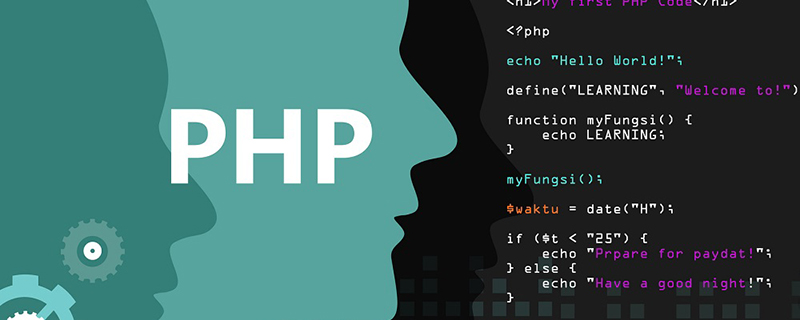• 本章节介绍一下如何删除一个字符串中重复字符，先不管有没有实际价值，就当做是一种对算法的学习也是挺不错的。 代码如下: function dropRepeat(str){ var result=[]; var hash={}; for(var i=0, elem; i<...
• （最简单的方法是把字符串看成一个字符数组，对该字符数组使用双重循环遍历，如果发现有重复的字符，就把该字符置为'\0',最后再把这个字符数组中所有的'\0'去掉，此时得到的字符串就是删除重复字符后的目标字符串） ...
预备知识：
0表示整数，'0'表示0字符，'\0'表示ASCII码值为0的字符
如果是 “字符串数组” 转 “字符串”，只能通过循环，没有其它方法
String[] str = {"abc", "bcd", "def"};
StringBuffer sb = new StringBuffer();
for(int i = 0; i < str.length; i++){
sb. append(str[i]);
}
String s = sb.toString();如果是 “字符数组” 转 “字符串” 可以通过下边的方法
String st=new String(c,0, l);  //第一个位置为字符数组名字，第二个为开始的位置，第三个为字符数组的长度
return st;1.蛮力法。（最简单的方法是把字符串看成一个字符数组，对该字符数组使用双重循环遍历，如果发现有重复的字符，就把该字符置为'\0',最后再把这个字符数组中所有的'\0'去掉，此时得到的字符串就是删除重复字符后的目标字符串）
public class Test {
public static void main(String args[]){
String str="abcaabcd";
str=removeDuplicate(str);
System.out.print(str);
}
private static String removeDuplicate(String str) {
char[] c=str.toCharArray();   //把字符串str转化为字符数组c[]
int len=c.length;
for(int i=0;i<len;i++){
if(c[i]=='\0')
continue;
for(int j=i+1;j<len;j++){
if(c[j]=='\0')
continue;
if(c[i]==c[j])
c[j]='\0';
}
}
int l=0;
for(int i=0;i<len;i++){      //得到每个元素只有一个的字符数组c[]
if(c[i]!='\0')
c[l++]=c[i];
}

System.out.println("l表示的是字符串的个数:"+l);
//将字符数组转化为字符串两种方法
/*
String st=new String(c,0, l);  //第一个位置为字符数组名字，第二个为开始的位置，第三个为字符数组的长度
return new String(st);
*/
return new String(c,0,l);  //第一个位置为字符数组名字，第二个为开始的位置，第三个为字符数组的长度
}
}



展开全文java
• 主要介绍了JS如何删除字符串中重复字符,需要的朋友可以参考下
• 利用php删除字符串中重复字符。比如：$str = "aaadddd"最后得到的应该是"ad"。思路：遍历整个数组，然后依次取出第i个字符，存入一个临时数组中，同时遍历临时数组，查找该数组中是否已经存在该字符。相关学习视频...利用php删除字符串中重复字符。比如：$str = "aaadddd"最后得到的应该是"ad"。思路：遍历整个数组，然后依次取出第i个字符，存入一个临时数组中，同时遍历临时数组，查找该数组中是否已经存在该字符。相关学习视频推荐：php视频教程代码如下：$b = 'aaaaaaaaaaaaddddddttttttffff';$tmp = array();for($i = 0;$i < strlen($b);$i  ){$len = count($tmp);for($j = 0;$j < $len;$j  ){if($b[$i] === $tmp[$j]){break;}}if($j ==$len){  //如果最后的$j和$len相等的话，则表示$tmp临时数组中不存在$b[$i]$tmp[] = $b[$i];}}$result = implode($tmp);//输出adtf相关文章教程推荐：php基础教程
展开全文• 最简单的方法就是把这个字符串看作是一个字符数组，对该数组使用双重循环进行遍历，如果发现有重复的字符，就把该字符置为’\0’，最后再把这个字符数组中所有的’\0’去掉，此时得到的字符串就是删除重复字符后的...
问题描述：

删除字符串中重复的字符，例如，”good”去掉重复的字符串后就变成”god”。

算法思路：

第一种方法：

“蛮力法”，最简单的方法就是把这个字符串看作是一个字符数组，对该数组使用双重循环进行遍历，如果发现有重复的字符，就把该字符置为’\0’，最后再把这个字符数组中所有的’\0’去掉，此时得到的字符串就是删除重复字符后的目标字符串。

第二种方法：

空间换时间，由于常见的字符只有256个，可以假设此题字符串不同的字符个数最多为256个，那么可以申请一个大小为256的int类型的数组纪录每个字符出现的次数，初始化都为0，把这个字符的编码作为数组的下标，在遍历字符数组时，如果这个字符出现的次数为0，那么把它置为1；如果这个字符出现的次数为1，说明这个字符在前面已经出现过了，可以把这个字符置为’\0’，就实现了去重的目的。这种方法只需要进行一次遍历，时间复杂度为O(n)，但是需要额外申请256大小的空间。

代码实现：

package JBString;

public class RemoveDuplicate {
public static String removeDuplicate(String str) {
char[] c = str.toCharArray();
int len = c.length;
for (int i = 0; i < len; i++) {
if (c[i] == '\0') {
continue;
}
for (int j = i + 1; j < len; j++) {
if (c[j] == '\0') {
continue;
}
// 把重复的字符置为'\0'
if (c[i] == c[j]) {
c[j] = '\0';
}
}
}
int l = 0;
// 去掉'\0'
for (int i = 0; i < len; i++) {
if(c[i] != '\0'){
c[l] = c[i];
l++;
}

}
return new String(c, 0, l);
}

public static String removeDaplicate(String str){
char[] c = str.toCharArray();
int len = c.length;
int[] flags = new int;   //只需要8个32bit的int
int i;
for (i = 0; i < 8; i++) {
flags[i] = 0;
}
for(i = 0; i < len; i++){
int index = (int)c[i]/32;
int shift = (int)c[i]%32;
if((flags[index] & 1 << shift) != 0){
c[i] = '\0';
}
flags[index] |= (1<<shift);
}
int l = 0;
for(i = 0; i < len; i++){
if(c[i] != '\0'){
c[l++] = c[i];
}
}
return new String(c,0,l);
}

public static void main(String[] args) {
String str = " abcaabcd";
str = removeDuplicate(str);
System.out.println(str);
}

}



展开全文空间换时间
• 1.将字符串中所有的重复元素的字符删除，只留下出现一次的字符 C语言是实现这个算法的过程有很多，比如说暴力拆解，新申请数组等等 今天小编给大家主要说说如何新申请数组简单理解这个算法 首先这个数组长度是不确定...
1.将字符串中所有的重复元素的字符删除，只留下出现一次的字符
C语言是实现这个算法的过程有很多，比如说暴力拆解，新申请数组等等
今天小编给大家主要说说如何新申请数组简单理解这个算法
首先这个数组长度是不确定的，并且中每个元素出现的次数很多很乱，如果但纯的遍历一遍数组就像排序一样，很容易出错，小编暴力拆解这个数组的时候首先数组移动真的是很麻烦而且这个元素重复出现基数次和偶数次是不一样的，所以说我就换了个算法。
首先我们需要一个动态整型数组和动态字符型数组，整型数组来记录没每个字符出现的次数，让这个出现的次数为1的时候将这个字符存放到新申请的动态数组中，用一个值来记录动态新字符型数组的长度，最后在新申请的数组末尾加上**’\0’**,这样一个新的数组就诞生了。
来看源码
#include"common.h"
//要求返回无重复字母数组
char* DeletePubChar(char* p)
{
int sz = (int)strlen§;//3
char* q = (char*)malloc(sizeof(char)(sz+1));//多申请一个内存空间存放‘\0’
int arr = (int*)malloc(sizeof(int)*sz);
memset(arr,0,sizeof(int)sz);
int i = 0;
int j = 0;
int k = 0;
for (i = 0; i < sz; ++i)
{
for (j = 0; j < sz; ++j)
{
if (p[i] == p[j])
arr[i] += 1;
}
if (arr[i] <= 1)
{
q[k] = p[i];
k++;
}
}
q[k + 1] = ‘\0’;
return q;
}
int main()
{
char arr[] = “abbascxxxx”;
charpp = DeletePubChar(arr);
printf("%s", pp);
system(“pause”);
return 0;
}
这样实现肯定时间空间复杂度是比暴力拆解高的，但是就是说稳定性很好。聪明的你有没有看明白你呢？


展开全文• ...say that i have string like this : <pre class="lang-php prettyprint-override"><code>$string = 'Life is like. . ....<pre class="lang-php prettyprint-override"><code>$string ='Life is like a box of ...php string
• <p>Imagine if: <pre><code>$string = "abcdabcdabcdabcdabcdabcdabcdabcd"; </code></pre> <p>How do I remove the repeated sequence of characters (all characters, not just alphabets) in the string so...php regex • 1. 已经排序好的字符串，将重复字符串保留一个 设两个标记：遍历标记和记录标记 遍历标记：pos 记录标记：index, 记录最终字符串的最后一个字节。 从第一个元素开始遍历，当前位置和有记录的位置不同，则拷贝... • 把字符串转换为字符数组，对数组进行双重循环遍历，如果发现有重复的字符，就把该字符置为'\0'，最后再把这个字符数组中的所有'\0'去掉，此时得到的字符串就是删除重复字符后的目标字符串。 方法二：正则表达式。用...Java • 利用php删除字符串中重复字符。比如：$str = "aaadddd"最后得到的应该是"ad"。思路：遍历整个数组，然后依次取出第i个字符，存入一个临时数组中，同时遍历临时数组，查找该数组中是否已经存在该字符。相关学习视频...
• package string.demos; import java.util.Hashtable; import java.util.Iterator... * 删除字符串中重复字符 * 方法： * ①“蛮力”法 * 把字符串看成一个字符数组，对该数组使用双重遍历， * 如果发现重...
• <p>Let's say i have the string $urlname = "pine-tree-tshirt--green-xs"</code></p> <p>I want my result to be$urlname = "pine-tree-tshirt-green-xs"</code></p> <p>I <strong>don't</strong> want to ...character duplicates php string
• 最简单的方法就是把这个字符串看作是一个字符数组，对该数组使用双重循环进行遍历，如果发现有重复的字符，就把该字符置为’\0’，最后再把这个字符数组中所有的’\0’去掉，此时得到的字符串就是删除重复字符后的...算法
• 在学习数组的相关知识后我开始尝试写一点有趣的东西来巩固自己学习的内容，所一我打算在本篇博客里分享一下如何利用操作数组并删除数组里重复的元素。 所用到的数组知识主要是数组的遍历，和数组的删除操作。 不说...Java
• 示例  face tell face <b> face </b>tool face   dlska sldkd face dlska <b> dlska </b>dldkf  可见<b>...里面的重复 ...如何删除<b>前面的词组</p>测试用例
• 如果我们有一个字符串，如'1122311223451'，我们想要去除其中重复出现的字符，并且保持每个字符第一次出现的次序，对于这个例子，它的结果就是'12345'，如何实现呢？ 方法可能有很多， 在这里推荐一种只需要一行...perl string
• p{1} = [1 2] p{2} = [1 2] p{3} = [3 4 5] ...这样的数据，可能会有很多，如何删除其中的重复项，不用循环判断，如何能高效完成 可用换一些方法转化为字符串，例如用sprintf: [~,k] = unique(cellfun(@(x)sp
• <p><strong>Possible Duplicate:</strong><br> <a href="https://stackoverflow.com/questions/1418193/how-to-get-file-name-from-full-path-with-php">How to get file name from full path with ...html php
• <p>This question already has an answer here: ... <a href="/questions/279170/utf-8-all-the-way-through" dir="ltr">UTF-8 all the way through ...<p>Actually I'm facing the problem while I fetch data...mysql php string
• <p>how to remove a duplicate word within a string , whereby this string is a value of an array ? <p>e.g <pre><code>foreach($results as$result) ... foreach($result as$words) ... echo \$words = str_...php
• 删除文件也会重复生成，非常痛苦。查阅crond相关日志，发现实际执行的内容为/lib/libudev.so ，以此为关键字进行查询，找到如下内容： 1.1 工具/原料 Linux系统 病毒文件libudev.so 1.2 方法/步骤 1....
• <p>This question already has an answer here: ... <a href="/questions/8163746/how-do-i-replace-certain-parts-of-my-string" dir="ltr">How do I replace certain parts of my string?...php
• 删除文件也会重复生成，非常痛苦。查阅crond相关日志，发现实际执行的内容为/lib/libudev.so ，以此为关键字进行查询，找到如下内容： 方法/步骤 1 1.網路流量暴增，使用 top 觀察有至少一個 10 個隨機...
• Possible Duplicate: 可能重复： To strip whitespaces inside a variable in PHP 在PHP中去除变量内的空格 How canphp string
• 删除字符串中的所有相邻重复项笔记删除字符串中的所有相邻重复项笔记思路代码Test结果如何改变文本的样式插入链接与图片如何插入一段漂亮的代码片生成一个适合你的列表创建一个表格设定内容居中、居左、居右...java leetcode 算法 数据结构
• <p>This question already has an answer here: ... <a href="/questions/1703320/remove-excess-whitespace-from-within-a-string" dir="ltr">Remove excess whitespace from within a string ...php
• 把字符串中的重复字符替换为空格。如何删除这些空格还没想好。 #include #include #include "stdafx.h" using namespace std; void removeDuplicate(char c[]){ char test; int i=0; int j=0; if(strlen......